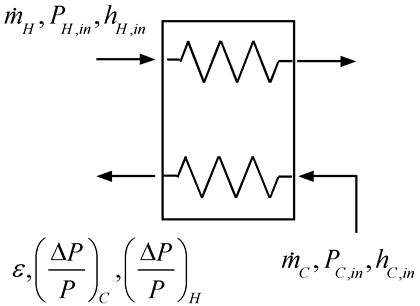Contents - index

HeatExchanger5_CLHeatExchanger5_CL uses the sub-heat exchanger model described by Nellis and Klein to simulate a recuperator.  The procedure works with any type of fluid other than 'AirH2O' and can deal with the situation where the pinch point in the heat exchanger occurs internally vs at the inlet or outlet.  Pressure drop, if specified, is applied linearly with heat transfer duty.  In this model the effectiveness is specified and the conductance and pinch point are computed.

Call HeatExchanger5_CL(F_H\$, C_H, P_H, h_H_in, m_dot_H, F_C\$, C_C, P_C, h_C_in, m_dot_C, eff, DPoverP_H, DPoverP_C, N: h_H_out, h_C_out, UA, DT_pp)

Inputs:

F_H\$:  String identifier for hot fluid

C_H:  concentration of hot fluid (%) (only used for a brine - otherwise set to 0)

P_H_in: Inlet pressure of hot fluid (bar, atm, Pa, kPa, or MPa depending on unitsystem)

h_H_in:  Inlet specific enthalpy of hot fluid (J/kg, kJ/kg, J/kmol, kJ/kmol, Btu/lbm, or Btu/lbmol depending on unit system)

m_dot_H: Mass (or molar) flow rate of hot fluid (kg/s, kmol/s, lbm/min, or lbmol/min, depending on unit system)

F_C\$:  String identifier for cold fluid

C_C:  concentration of cold fluid (%) (only used for a brine - otherwise set to 0)

P_C_in: Inlet pressure of cold fluid (bar, atm, Pa, kPa, or MPa depending on unitsystem)

h_C_in:  Inlet specific enthalpy of cold fluid (J/kg, kJ/kg, J/kmol, kJ/kmol, Btu/lbm, or Btu/lbmol depending on unit system)

m_dot_C: Mass (or molar) flow rate of cold fluid (kg/s, kmol/s, lbm/min, or lbmol/min, depending on unit system)

eff: Effectiveness of heat exchanger = Q_dot/Q_dot_max where Q_dot_max occurs if pinch point temperature difference is 0

DPoverP_H: Pressure drop in hot fluid expressed as a fraction of the inlet pressure

DPoverP_C: Pressure drop in cold fluid expressed as a fraction of the inlet pressure

N: Number of sub heat exchangers (if set <1 then default value of N=10 is used)

Outputs:

h_H_out:  Outlet specific enthalpy of hot fluid (J/kg, kJ/kg, J/kmol, kJ/kmol, Btu/lbm, or Btu/lbmol depending on unit system)

h_C_out:  Outlet specific enthalpy of cold fluid (J/kg, kJ/kg, J/kmol, kJ/kmol, Btu/lbm, or Btu/lbmol depending on unit system)

UA: Conductance of heat exchanger (W/K or Btu/hr-R, depending on unit system)

DT_pp: Pinch point temperature difference (K)

Example:

\$UnitSystem SI Mass J K Pa

F_H\$='CarbonDioxide'

P_H = 20 [MPa]*convert(MPa,Pa)

T_H_in = converttemp(C,K,700 [C])

h_H_in = enthalpy(F_H\$,P=P_H,T=T_H_in)

m_dot_H = 1 [kg/s]

F_C\$='CO2'

P_C = 7 [MPa]*convert(MPa,Pa)

T_C_in = converttemp(C,K,40 [C])

h_C_in = enthalpy(F_C\$, T=T_C_in)

m_dot_C = 4.5 [kg/s]

DPoverP_H = 0.05

DPoverP_C = 0.05

N = 10 [-]

eff = 0.8

Call HeatExchanger5_CL(F_H\$, 0 [%], P_H, h_H_in, m_dot_H, F_C\$, 0 [%], P_C, h_C_in, m_dot_C, eff, DPoverP_H, DPoverP_C, N: h_H_out, h_C_out, UA, DT_pp)

{Solution:

h_H_out = -39514 [J/kg]

h_C_out = -8.760e6 [J/kg]

UA = 3745 [W/K]

DT_pp = 76.15 [K]}